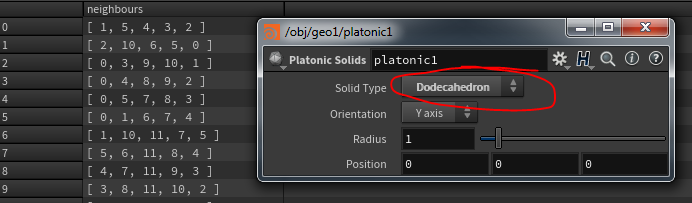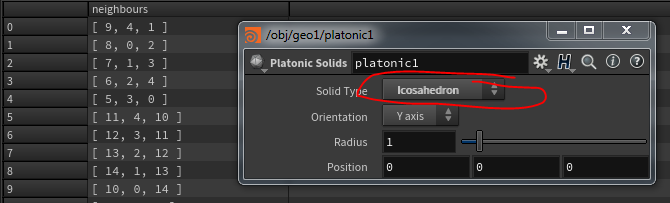Jump to content

## Recommended Posts

Looks like you were on the right track with the use of half edges. You could approach the problem using a primitive wrangle as follows:

```// Array of neighbouring prims
int prim_neighbours[];

// Loop through the primitives vertices
foreach (int vtx; primvertices(0, @primnum))
{
// Get the forward half edge for the vertex
int this_hedge = vertexhedge(0, vtx);

// Only process if a valid half edge
if (hedge_isvalid(0, this_hedge))
{
int equiv_hedge = this_hedge;

// Loop through equivalent half edges and get the neighbouring prims
do
{
equiv_hedge = hedge_nextequiv(0, equiv_hedge);

if (equiv_hedge != this_hedge)
{
// !! Get your attribues here !!
push(prim_neighbours, hedge_prim(0, equiv_hedge));
}

} while (equiv_hedge != this_hedge);
}
}

i[]@neighbours = prim_neighbours;```

Edited by Wooshum
Code mistake
•1

#### Share this post

##### Share on other sites

another way:

```//primwrangle

int pt = addpoint(0, @P); //center for each primitive
removeprim(0, @primnum, 1); //we just want the new created points```

connect adjacent pieces with type set to adj. points. set radius to correct value to not get too many connections. unit icosahedron would be 0.3 for example

```//pointwrangle

int nb[] = neighbours(0, @ptnum); //this array is the neighours for each point. point numbers correspond to prim numbers```

#### Share this post

##### Share on other sites

Dan, one more question. It seems that now I have a list of points and a list of primitives with 5 attributes instead of three.  I guess it is because I found the adjacent points. How can I create a list with the adjacent primitives - triangles?

#### Share this post

##### Share on other sites

The number of neigbouring primitives returned is dependent on the number of edges each primitive (as in face/polygon) shares with another primitive. So in your example a Dodecahedron will return 5 neighbours per prim as each face has 5 shared edges. If you start with a Icosahedron you will return 3 neighbours per prim, etc.Primitives in houdini are n-sided polygons so can have any number of shared edges.

## Create an account or sign in to comment

You need to be a member in order to leave a comment

## Create an account

Sign up for a new account in our community. It's easy!

Register a new account

## Sign in

Already have an account? Sign in here.

Sign In Now

×
• Donations

• Leaderboard